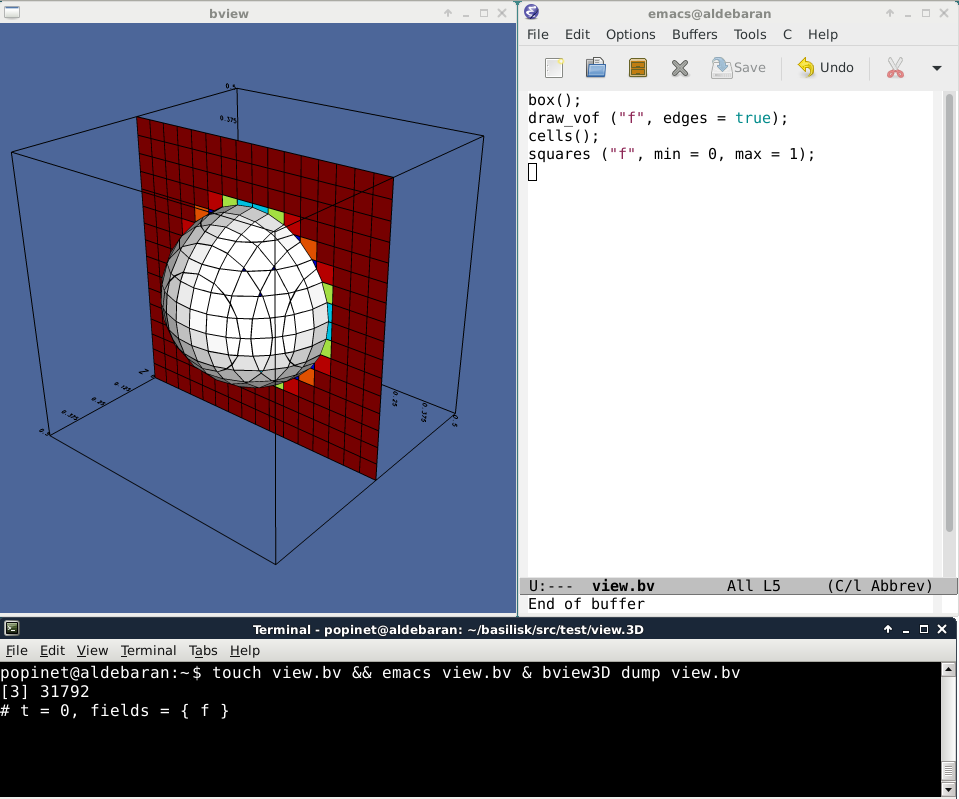Interactive Basilisk View

This shell script provides a simple way to start the Interactive Basilisk View interface.

Note that two versions of the script are provided depending on the number of dimensions: bview2D and bview3D.

The server bview-server.c can be run on the local machine or on a distant server. The client bview-client.py runs on the local machine and provides graphical display and interaction.

The server’s input pipe is connected both to the graphical interface of the client and to the standard input on the local machine, so that commands can be sent to the server either through the GUI or by typing drawing commands on standard input.

Command-line arguments

Basilisk dump files or view files (with extension .bv) can be given as command-line arguments.

If a .bv file is given, the script also watches for any modifications of this file. If changes are made, the current view is cleared and the file is reloaded. Editing this file with a text editor thus gives another way of interacting with the server.

Dump files are also watched for any modifications. This can be used to automatically update the display of a running simulation.

Installation

Note that the servers need to be installed separately before using bview.

You also need to check that the dependencies of the Python client are verified.

Examples

A typical session could look like:

popinet@aldebaran:~$bview3D restore ("dump"); # t = 0.01, fields = { u.x u.y u.z g.x g.y g.z f rhov } box (); draw_vof ("f"); clear (); box (); squares ("f", min = 0, max = 1); show (); view (fov = 24, quat = {-0.242493,0.267956,0.0538964,0.930855}, \ tx = 0, ty = 0, bg = {0.3,0.4,0.6}, width = 800, height = 800, samples = 4); box (); squares ("f", min = 0, max = 1); save ("view.bv"); or popinet@aldebaran~:$ touch view.bv && emacs view.bv & bview3D dump view.bv
# t = 0.01, fields = { u.x u.y u.z g.x g.y g.z f rhov }

Followed by editing (and saving) the view.bv file.A typical bview session.

Implementation

dimension=echo -n $0 | tail -c 2 watched="" watchedd="" while test$# -gt 0; do
case $1 in *@*) remote=$1 ;;
*.bv) watched="$watched$1"; args="$args$1" ;;
*) watchedd="$watched$1"; args="$args$1" ;;
esac
shift
done

fifo=mktemp -u -t bview.XXXXXX
mkfifo $fifo if test -z "$remote"; then
bview-server$dimension$args < $fifo | \ python2.7$BASILISK/bview-client.py > $fifo & else ssh$remote bview-server$dimension$args < $fifo | \ python2.7$BASILISK/bview-client.py > $fifo & fi pids=$!

function ctrl_c() {
rm -f $fifo kill$pids %1 $(jobs -p) 2> /dev/null } trap 'ctrl_c' SIGINT modtime() { if test -f$1; then
date -r $1 +%s fi } watchbv() { time=modtime$1
while true; do
time1=modtime $1 if [[ "$time1" != "$time" ]] ; then echo "interactive (false);" echo "clear();" cat$1
echo
echo "interactive (true);"
echo "display();"
time="$time1" fi sleep 1 done } watchdump() { time=modtime$1
while true; do
time1=modtime $1 if [[ "$time1" != "$time" ]] ; then echo "restore (\"$1\");"
time="$time1" fi sleep 1 done } for f in$watched; do
watchbv $f >$fifo &
done

for f in $watchedd; do watchdump$f > $fifo & done cat >$fifo

ctrl_c